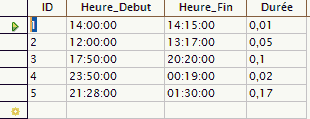# Faq/Base/120

< Faq‎ | Base

Other languages:
dansk • ‎Deutsch • ‎English • ‎español • ‎français • ‎italiano

## How can I calculate a time?

Starting with two fields, one for starting time the other for finishing time, the difference between them in minutes can be calculated with a query like the following:

`SELECT "ID", "Heure_Debut", "Heure_Fin" CAST('00:' || DATEDIFF ('mi',"Heure_Debut","Heure_Fin) || ':00' AS "TIME") AS "Durée" FROM  "Table1"`

Explanation• The DATEDIFF function can be used to calculate the number of minutes between two times.
• The operator || concatenates the result with strings of zeroes representing hours and seconds. An example result would be '00:75:00'.
• CAST converts this string to time format (TIME).
• Internally a date or time is a numerical value, '1' corresponds to one day. The integer part represents the date and the fractional part the time.
• By default the column header is the formula used for the calculation. It is usually preferable to add an alias (Durée in this example)

To get the result in time format, use the function TO_CHAR to format it.

`SELECT "ID","Heure_Debut","Heure_Fin" TO_CHAR(CAST( '00:'|| DATEDIFF( 'mi',"Heure_Debut","Heure_Fin)||':00' AS "TIME" ), 'HH:MI') AS "Durée" FROM "Table1"`